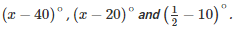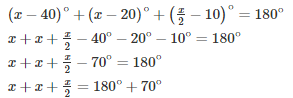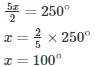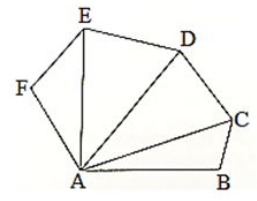Courses

# RD Sharma Solutions - Ex-15.2, (Part - 1), Properties Of Triangles, Class 7, Math Class 7 Notes | EduRev

## RD Sharma Solutions for Class 7 Mathematics

Created by: Abhishek Kapoor

## Class 7 : RD Sharma Solutions - Ex-15.2, (Part - 1), Properties Of Triangles, Class 7, Math Class 7 Notes | EduRev

The document RD Sharma Solutions - Ex-15.2, (Part - 1), Properties Of Triangles, Class 7, Math Class 7 Notes | EduRev is a part of the Class 7 Course RD Sharma Solutions for Class 7 Mathematics.
All you need of Class 7 at this link: Class 7

Q1. Two angles of a triangle are of measures 150 and 30. Find the measure of the third angle.

Solution.

Let the third angle be x

Sum of all the angles of a triangle = 180

105+ 30+x = 180

135+ x = 180

x = 180∘ – 135

x = 45

Therefore the third angle is 45

Q2. One of the angles of a triangle is 130, and the other two angles are equal What is the measure of each of these equal angles?

Solution.

Let the second and third angle be x

Sum of all the angles of a triangle = 180

130∘+ x+x = 180

130+2x = 180

2x = 180– 130

2x = 50

x = 50/2

x = 25

Therefore the two other angles are 25 each

Q3. The three angles of a triangle are equal to one another. What is the measure of each of the angles?

Solution.

Let the each angle be x

Sum of all the angles of a triangle = 180

x+x+x = 180

3x = 180

x = 1803

x = 60

Therefore angle is 60 each

Q4. If the angles of a triangle are in the ratio 1 : 2 : 3, determine three angles.

Solution.

If angles of the triangle are in the ratio 1:2:3 then take first angle as ‘x’, second angle as ‘2x’ and third angle as ‘3x’

Sum of all the angles of a triangle = 180

x+2x+3x = 180

6x = 180

x = 180/6

x=30

2x = 30×2 = 60

3x = 30×3 = 90

Therefore the first angle is 30, second angle is 60 and third angle is 90

Q5. The angles of a triangle areFind the value of x.

Solution.

Sum of all the angles of a triangle = 180Hence we can conclude that x is equal to 100

Q6. The angles of a triangle are arranged in ascending order of magnitude. If the difference between two consecutive angles is 10. Find the three angles.

Solution.

Let the first angle be x

Second angle be x+10

Third angle be x+10+ 10

Sum of all the angles of a triangle = 180

x+ x+10+ x+10+ 10 = 180

3x+30 = 180

3x = 180-30

3x = 150

x = 150/3

x = 50

First angle is 50

Second angle x+10 = 50+10 = 60

Third angle x+10+ 10 = 50+10+10 = 70

Q7. Two angles of a triangle are equal and the third angle is greater than each of those angles by 30. Determine all the angles of the triangle

Solution.

Let the first and second angle be x

The third angle is greater than the first and second by 30 = x+30

The first and the second angles are equal

Sum of all the angles of a triangle=180∘

x+x+x+30 = 180

3x+30 = 180

3x=180-30

3x = 150

x = 150/3

x = 50

Third angle = x+30 = 50+ 30 = 80

The first and the second angle is 50 and the third angle is 80

Q8. If one angle of a triangle is equal to the sum of the other two, show that the triangle is a right triangle.

Solution.

One angle of a triangle is equal to the sum of the other two

x = y+z

Let the measure of angles be x,y,z

x+y+z = 180

x+x = 180

2x = 180

x = 180/2

x = 90

If one angle is 90 then the given triangle is a right angled triangle

Q9. If each angle of a triangle is less than the sum of the other two, show that the triangle is acute angled.

Solution.

Each angle of a triangle is less than the sum of the other two

Measure of angles be x,y and z

x>y+z

y<x+z

z<x+y

Therefore triangle is an acute triangle

Q10. In each of the following, the measures of three angles are given. State in which cases the angles can possibly be those of a triangle:

(i) 63, 37, 80

(ii) 45, 61, 73

(iii) 59, 72, 61

(iv) 45, 45, 90

(v) 30, 20, 125

Solution.

(i) 63, 37, 80 = 180

Angles form a triangle

(ii) 45, 61, 73 is not equal to 180

Therefore not a triangle

(iii) 59, 72, 61 is not equal to 180

Therefore not a triangle

(iv) 45, 45, 90 = 180

Angles form a triangle

(v) 30, 20, 125 is not equal to 180

Therefore not a triangle

Q11. The angles of a triangle are in the ratio 3: 4 : 5. Find the smallest angle

Solution.

Given  that

Angles of a triangle are in the ratio: 3: 4: 5

Measure of the angles be 3x, 4x, 5x

Sum of  the angles of a triangle = 180

3x+4x+5x = 180

12x = 180

x = 180/12

x = 15

Smallest angle = 3x

= 3 x 15

= 45

Q12. Two acute angles of a right triangle are equal. Find the two angles.

Solution.

Given acute angles of a right angled triangle are equal

Right triangle: whose one of the angle is a right angle

Measured angle be x,x,90

x+x+180 = 180

2x = 90

x = 90/2

x = 45

The two angles are 45  and 45

Q13. One angle of a triangle is greater than the sum of the other two. What can you say about the measure of this angle? What type of a triangle is this?

Solution.

Angle of a triangle is greater than the sum of the other two

Measure of the angles be x,y,z

x>y+z   or

y>x+z  or

z>x+y

x or y or z>90 which is obtuse

Therefore triangle is an obtuse angle

Q14. AC, AD and AE are joined. Find ∠FAB+∠ABC+∠BCD+∠CDE+∠DEF+∠EFA

Solution.∠FAB+∠ABC+∠BCD+∠CDE+∠DEF+∠EFA

We know that sum of the angles of a triangle is 180

Therefore in ΔABC,we have

∠CAB+∠ABC+∠BCA = 180—(i)

In ΔACD,we have

∠DAC+∠ACD+∠CDA = 180—(ii)

In ΔAEF,we have

∠FAE+∠AEF+∠EFA = 180—(iv)

Therefore

∠FAB+∠ABC+∠BCD+∠CDE+∠DEF+∠EFA = 720

97 docs

,

,

,

,

,

,

,

,

,

,

,

,

,

,

,

,

,

,

,

,

,

,

,

,

,

,

,

,

,

,

,

,

,

;